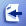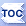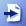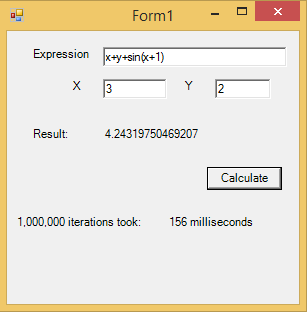Above: Visual Basic Demo ApplicationbcParser.NET is a mathematical expression parser library for the .NET platform.

bcParser.NET math parser library parses and evaluates mathematical expressions given as strings at runtime. String formula can contain user defined variables and functions.

The C# Class that implements the parser is Bestcode.MathParser.MathParser.  It was tested with Visual Studio 2003, 2005 and 2008, 2010, 2013, 2015 and 2019. bcParser.NET can be used with 32 and 64 bit Windows as well as with DotNet Distributions for Mac OS and Linux. .NET Core is supported.

You can download the evaluation version which includes C# sample, VB sample and a time limited evaluation DLL. There is also a demo VB.NET application.

You can view the html documentation of bcParser.NET. (MathParser class)

# Math Parser for .NET, C#, Visual Basic

bcParser.NET math parser library features include:

• Easy to use, simple class API.
• Functions with 0 or more number of parameters in the form of: f(x,y,z, ...)
• Functions with unknown number of parameters, e.g. SUM(a,b,c,...)
• Function parameter calculations are only done if needed.
• Comes with predefined functions.
• You can create custom functions/variables with callbacks to the functions that you define in your source code, either through delegates or interfaces.
• VariableDelegate to provide values for undefined variables.
• Function/variable names start with letters and can contain letters, numbers and _.
• Expression can contain string literals, variable values and function return values can be strings.
• Arithmetic Operators: +, -, /, *, ^
• Boolean Operators: <, >, =, &, |, ! ,<>, >=, <=
• String concatenation with & or +
• Optimization: Constant expression elimination for repeated tasks.
• Paranthesis: (, {, [
• List of predefined functions is available in the documentation.
• Provides localization support.
• Optional culture specific decimal separator support (dot or comma).
• Double or Decimal type support by conditional compilation (Shipped binaries are compiled for double type by default, you need to recompile for decimal type).
• Royalty free distribution.
• Source code is included.

bcParser.NET mathematical expresison parser is especially useful in scientific and engineering programs as well as financial spread sheet implementations. To support financial needs, bcParser.NET provides a conditional compilation option to use Decimal type instead of double floating point numbers (You need to set the compiler directive and rebuild it for Decimal support - trial version does not have this).

• C# Example
• Visual Basic Example
• Delphi.NET Example
• List of predefined functions
• Build the source - FAQ

• #### The Math Parser Component is also available on many platforms/languages such as:

Examples of typical mathematical formulas are:

 CELL(F1)+(X-STRLEN(CELL(A 1)+*)) String literals, functions, variables and constants are supported. This makes it possible to simulate variables that are not defined ahead of time. For example, you may have a database (or a spreadsheet) that you retrieve values from, but you do not know which table or column the user will use (so you cant predefine variables with those names). You could allow: LN(X)+VALUE(MYTABLE,MYCOLU MN) or spreadsheet related expression could look like CELL(F1) for example, instead of defining a variable called F1. SIN(3.14)+5^2+POW(2,MAX(X* 2,Y)) Functions, variables, constants can be nested.Common math functions are defined by default. 2*[LN(1+X) / LOG(1-X)] Paranthesis can be (, {, [ for readability. IF(X>0, 3/X, F(X)) You can avoid Division By Zero errors as in 3/X.(3/X will not be evaluated if X>0 is false - parameters to functions are evaluated only if required by the internal logic of a function). IF(a,b,c) branching function is supported. Boolean operators are supported. Any non-zero value is TRUE, 0 is FALSE. Functions can be defined to have 0,1,2,...N numbers of parameters, or unknown number of parameters (whatever the user passes will be the parameters). PI*(R^2) Constants supported. For example, PI is a constant, not a variable. When optimization is turned on, constants may improve the speed of repeated evaluations where expression does not change but variable values change. X+Y/LOG(1+5) If Optimization is turned ON, LOG(1+5) will be optimized away since it is a constant. VOLUME(HEIGHT, WIDTH, LENGTH) You can create your own functions and variables and name them as you wish. You can replace a predefined function with your own implementation. RND() Functions with 0 parameters are supported. SUM(1,2,3,4,5,6,...n) Functions that take unknown number of parameters are supported. X+COSH(3E-2) Scientific notation is supported: 3E-2To be efficient in repeated calculations, parser compiles the expressionfirst and re-uses the resulting abstract syntax tree for each evaluation without the need to reparse.

Optimizer: If Optimization is on, the parsed tree structure will be optimized by calculating constant expression sections at once so that further evaluation requests will be quicker without the need to re-evaluate those constant branches.

bcParser.NET comes with the C# source code and there is also help documentation available for reference. Download is a single zip file with size of around 500KB. Package contains source files, bcParser.NET.DLL file, sample C# and sample VB.NET applications, HTML Documentation which is also available online.

Here is a short code snippet (C# Example) a VB Example and a Delphi.NET example to help you get started using bcParser.NET. Release version comes with full C# source code.

Here is an example of using bcParser.NET library with .NET Core on Linux with VSCode: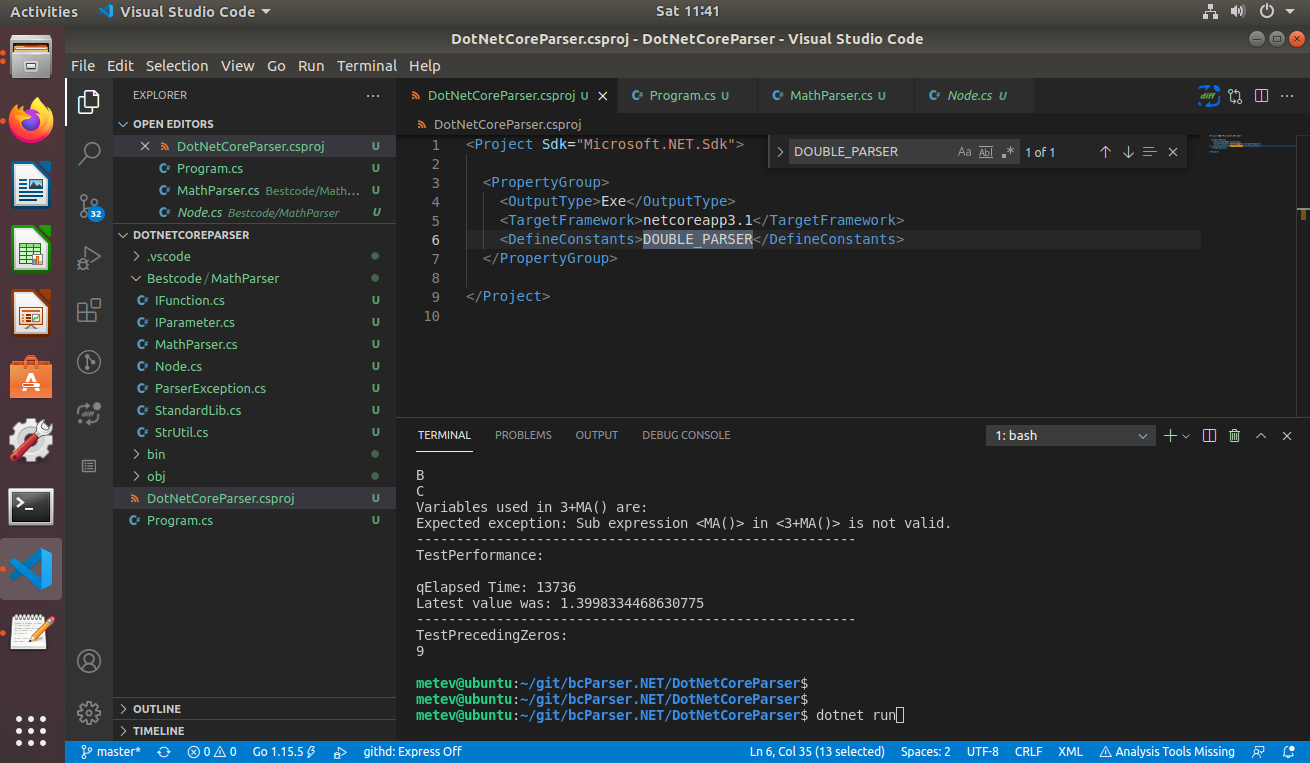## Purchasing bcParser.NET Math Parser Component

You can pay with credit card and download bcParser.NET immediately from our online store for only \$29.95. Full version includes C# source code. Upgrades are free for registered users. Licensing is per developer. Site license option is available in the online store page. You can deploy the the component royalty free with your applications as many times as you want. Site license allows any number of developers use the component at your development site. Site License is \$290.95. Site licenses can be purchased here.